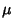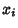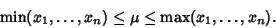## Mean

A mean is Homogeneous and has the property that a meanof a set of numberssatisfiesThere are several statistical quantities called means, e.g., Arithmetic-Geometric Mean, Geometric Mean, Harmonic Mean, Quadratic Mean, Root-Mean-Square. However, the quantity referred to as the'' mean is the Arithmetic Mean, also called the Average.